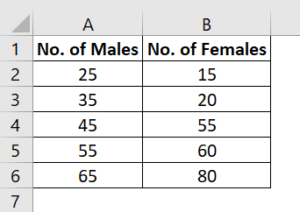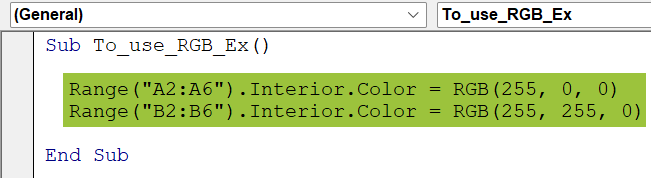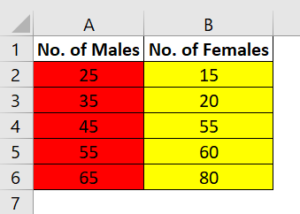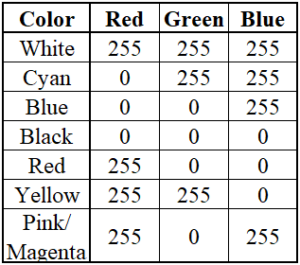# VBA RGBArticle byExcelMojo Team## What is Excel VBA RGB Color?

VBA RGB represents the red, green, and blue color options in VBA Excel. With the help of VBA RGB color, we can change the color of the font or a cell, or both. VBA RGB is the dedicated function to utilize the colors red, green, and blue while coding. All other colors are their components ranging from 0 to 255.

Let us understand this function in VBA with a simple example. Suppose we want to change the font color of a cell using the VBA RGB function. Please follow the below steps. First, write the data in the Excel sheet shown below. Here, we have written the days of the week in black. Next, write the below code in a module in Visual Basic in the Developer tab in excel.  Finally, run the code by clicking the Run option in the VBA ribbon. You can see the font color change to magenta (255,0,255).

Code:

Sub VBA_RGB_Ex()
Range(“A1:A10”).Font.Color = RGB(255, 0, 255)
End Sub

###### Key Takeaways
• The VBA RGB function represents the red, green, and blue colors. It is used to set the font colors or cells in Excel worksheets.
• The syntax to set any color is as follows:
• The VBA RGB function has three arguments of type Integer. The value ranges from 0 to 255 for all three.
• The function returns the color code value as a Long data type. Color constants can also be used in the VBA code instead of actual values.
•  The VBA RGB color picker function sets RGB as a default color palette. We can easily pick a suitable color from the palette.

### How to Change the Color of Cells Using VBA RGB Function?

Here we are going to change the color of some cells (Interior color) using VBA RGB Function.

1. We have two columns for the number of males and females in classes in a school.2. Open a new module and write the below code:

Sub To_use_RGB_Ex()
Range(“A2:A6”).Interior.Color = RGB(255, 0, 0)
Range(“B2:B6”).Interior.Color = RGB(255, 255, 0)
End Sub

• Explanation:
Range(“A2:A6”).Interior.Color = RGB(255, 0, 0): This code is used to color the interior of cell range A2-A6 with the red color (255, 0, 0).

Range(“B2:B6”).Interior.Color = RGB(255, 255, 0): This adds yellow (255, 255, 0)to the cell range B2-B6.3. Run the code and we can see the color change as red for the cell range A2:A6 and yellow for the cell range B2:B6.### Examples

Let us look at some examples where we can use the RGB function to color objects in Excel.

#### Example #1

Here, we will see the use of the RGB function in coloring the font as well as the cell via VBA code.

• Step 1: We have the following data in an Excel sheet.
• Step 2: Open the VBA module in the Developer Tab under Visual Basic and write the below code:

Code:

Sub VBA_RGB_Ex()
Range(“A1:A5”).Font.Color = RGB(255, 0, 255)
Range(“A1:A5”).Interior.Color = RGB(0, 0, 255)
End Sub

• Explanation:

Range(“A1:A5”).Font.Color = RGB(255, 0, 255)- It will give a magenta color to the font value in the cell range A1-A5.

Range(“A1:A5”).Interior.Color = RGB(0, 0, 255)-It will assign a blue color to the interior of the cells in A1-A5.

• Step 3: Run the code by clicking the Run button within the VBA ribbon or F5. We can see the below result in our worksheet.

#### Example #2

In this example, we will use the RGB function to create a combination of colors in the Excel cells. Here, we will also see the steps to obtain the value of any color using the VBA code.

• Step 1: Write the below code as below.

This code shows the value of red (VBA.ColorConstants.vbRed) in cell A1.

• Step 2: Here, the font color of the A3 cell value is assigned the red color.
• Step 3: This line assigns the value “RED” to cell A3. Cell A2 is assigned an interior color of red.
• Step 4: We write the rest of the code, assigning different colors to the font, cell, and other values to cells B1:B3, C1:C3, and D1:D3.

The above code is used to assign a combination of font colors, text, and cell colors to the D1-D3 cells.

Code:

Sub Using_RGB_Ex()
Range(“A1”).Value = VBA.ColorConstants.vbRed
Range(“A2”).Interior.Color = vbRed
Range(“A3”).Font.Color = vbRed
Range(“A3”).Value = “RED”

Range(“B1”).Value = VBA.ColorConstants.vbGreen
Range(“B2”).Interior.Color = vbGreen
Range(“B3”).Font.Color = vbGreen
Range(“B3”).Value = “Green”

Range(“C1”).Value = VBA.ColorConstants.vbCyan
Range(“C2”).Interior.Color = vbCyan
Range(“C3”).Font.Color = vbCyan
Range(“C3”).Value = “Cyan”

Range(“D1”).Value = VBA.ColorConstants.vbGreen + vbRed
Range(“D2”).Interior.Color = vbGreen + vbRed
Range(“D3”).Font.Color = vbGreen + vbRed
Range(“D3”).Value = “Yellow”
End Sub

• Step 5: Run the code by clicking the Run button within the VBA ribbon, and you can see the below result in your worksheet.

### Important Things to Note

• VBA RGB can assign any color or combination using VBA code using VBA code to obtain different shades of red, green, and blue. For example, cyan color is a mix of red(68), green(247), and blue(255).
• The value of the colors is available between 0 to 255 only. If the value exceeds 255, it will reset it to 255.
• We can also find the value of a color using the VBA code.
• The VBA.ColorConstants is used to assign different colors. It has eight color options.
• The input of the Color property is two types:
• vbColor
• RGB colors
• vbColors is the easiest way of setting colors in the VBA. However, the color options are minimal.
• RGB has three primary colors, which are combined to give multiple colors.
• VBA RGB function is beneficial in representing the data, as the process of the color change of fonts or cells can be automated.

1. What is the RGB for the green in VBA?

The value of RGB for green in VBA is RGB(0,255,0). This can be used to color a cell or change the font color of a value in a cell in VBA.

2. What is the RGB color range in VBA?

In VBA, every color is defined by a value. Fifty-six shades are available for RGB, ranging from 0 to 255. Below is a table representing some popular colors.3. Why is RGB in VBA not working?

If VBA RGB is not working in Excel, add or update the custom RGB color into the MS Excel color palette. There are minimal options in the older version of Excel (.xls, 2003). However, the advanced versions have no such limitation (.xlsx, 2010-11). You can change or add color using the Workbook.changePalette method.